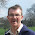## Saturday, April 5, 2014

### Modeling an On/Off Span

I may be ruining a perfectly good homework problem by posting this. :-)

Occasionally someone needs to incorporate in an integer programming model the notion of something changing state for a predefined span of time. The typical characterization I've seen is as follows:
• we have a sequence of binary variables $x_i\in\{0, 1\}, i\in\{1,\dots,N\}$ that indicate the state of something; and
• if the state changes from 0 to 1, it must remain 1 for exactly $K$ consecutive periods (with a possible exception of we reach the horizon limit $N$ before we see the $K$-th unit value).
For $K=3$, this means the sequence <... 0 1 1 1 0 ...> is valid but <... 0 1 1 0 ...> is invalid (not enough ones), <... 0 1 1 1 1 0 ...> is invalid (too many ones), and <... 0 1 1> (bumping into the end of the horizon) may or may not be considered valid. The question is, how do we model this with binary variables.

The answer is to rethink our choice of variables: rather than focusing on binary variables representing the system state, we focus on binary decision variables indicating whether or not a particular epoch represents the start of a string of ones. So we introduce a new set of binary variables $y_i\in\{0, 1\}, i\in\{1,\dots,N\}$, where $y_i=1$ if and only if a string of $x$ variables starting at epoch $i$ take the value 1. We enforce all this with the following constraints:
1. $x_{i-1}\le 1-y_i, i\in\{2,\dots,N\}$ (the value of $x$ in the epoch immediately prior to the start of a run must be 0);
2. $x_{i+K}\le 1-y_i, i\in\{1,\dots,N-K\}$ (the value of $x$ in the epoch immediately after the end of a run must be 0); and
3. either $$x_i\ge y_j \forall i\in\{1,\dots,N\}, \forall j\in \{i-K+1,\dots,i\}$$ or $$x_i\ge \sum_{j=i-K+1}^i y_j,$$eliminating any terms with indices outside the domain $\{1,\dots,N\}$ (the $K$ values at or immediately after the start of a run must be 1).
The second version of (3) is more compact (good) but makes the constraint  matrix denser (not so good). I'm pretty sure the second version produces the tighter feasible region.

If you do not want the time frame to end on a run of fewer than $K$ ones, constrain $y_j=0$ for $j\in \{N-K+2,\dots,N\}$. If you must have at least one zero after the final run of ones (i.e., <... 0 1 1 1 0> is okay for $K=3$ but <... 0 1 1 1> is not), constrain $y_j=0$ for $j\in \{N-K+1,\dots,N\}$. If you do not want to start a run of ones at the very beginning of the time frame (<0 1 1 1 0 ...> is okay but <1 1 1 0 ...> is not), constrain $y_1=0$.

1.Thank you Paul for this post; as you say, it may spoil a homework problem, but my experience was that some mathematicians find the concept of zero-one variables extremely difficult. When I taught IP, I used several examples like this in my lectures and notes, but found that few students could "invent" a formulation that was different from those they had seen in class, and certainly not under examination conditions. It would be interesting if anyone has found a way to teach this dark art of binary variable modelling!

2.Thanks David. You raise an important point about teaching how to model with binary variables. I do not recall ever finding a good (and thorough) exposition suitable for classroom use. To the extent it was covered at all in texts, it was mainly "stock" problems (such as shortest path, bin packing or TSP), which fuels the problem you note of students being able to reproduce only those models they have previously seen. Beyond those standard models, exposition tends to be anecdotal ("here is the model we used to solve problem X"), and more in journal articles than in books.

The best exposition I have seen comes from one of your countrymen. "Model Building in Mathematical Programming" by H. Paul Williams (Dept. of OR, London School of Economics) has, in addition to copious examples, a nice section on "Logical Conditions and Zero-One Variables".

One of my pet peeves is that textbook authors, when they do venture in binary decision variables, throw up "Big M" formulations without (a) subscripting M or (b) discussing issues of tightness of the formulation or numerical instability. This leads to users thinking they need just one "M" for all logical constraints, and that it should be really, really large ... which leads to really, really slow progress and in some cases really, really incorrect answers. Even Prof. Williams falls a bit short in this regard.

3.The Big M constraints is probably the first place where preprocessing of MIP solvers will adjust some coefficients. When I started doing MIPs as an undergrad, we had plenty of problems with big Ms. In my supply chain class, some students used very high values of "M" in models and CPLEX didn't break a sweat. It's still good to know what to do rather than rely on the solver to correct one's mistakes, however.

1.Marc-Andre: It does help that solvers may reduce M during preprocessing, but I'm not sure how much I would rely on that. Some years ago, I attended a talk by Ed Klotz in which he discussed if-then constraints (a relative recent addition to CPLEX at the time). Internally they are (usually?) implemented as big-M constraints, with CPLEX picking a value of M. I recall Ed saying that if the user knew a good value for M, he or she was probably better off using that value than relying on CPLEX to pick one.

Due to intermittent spamming, comments are being moderated. If this is your first time commenting on the blog, please read the Ground Rules for Comments. In particular, if you want to ask an operations research-related question not relevant to this post, consider asking it on Operations Research Stack Exchange.